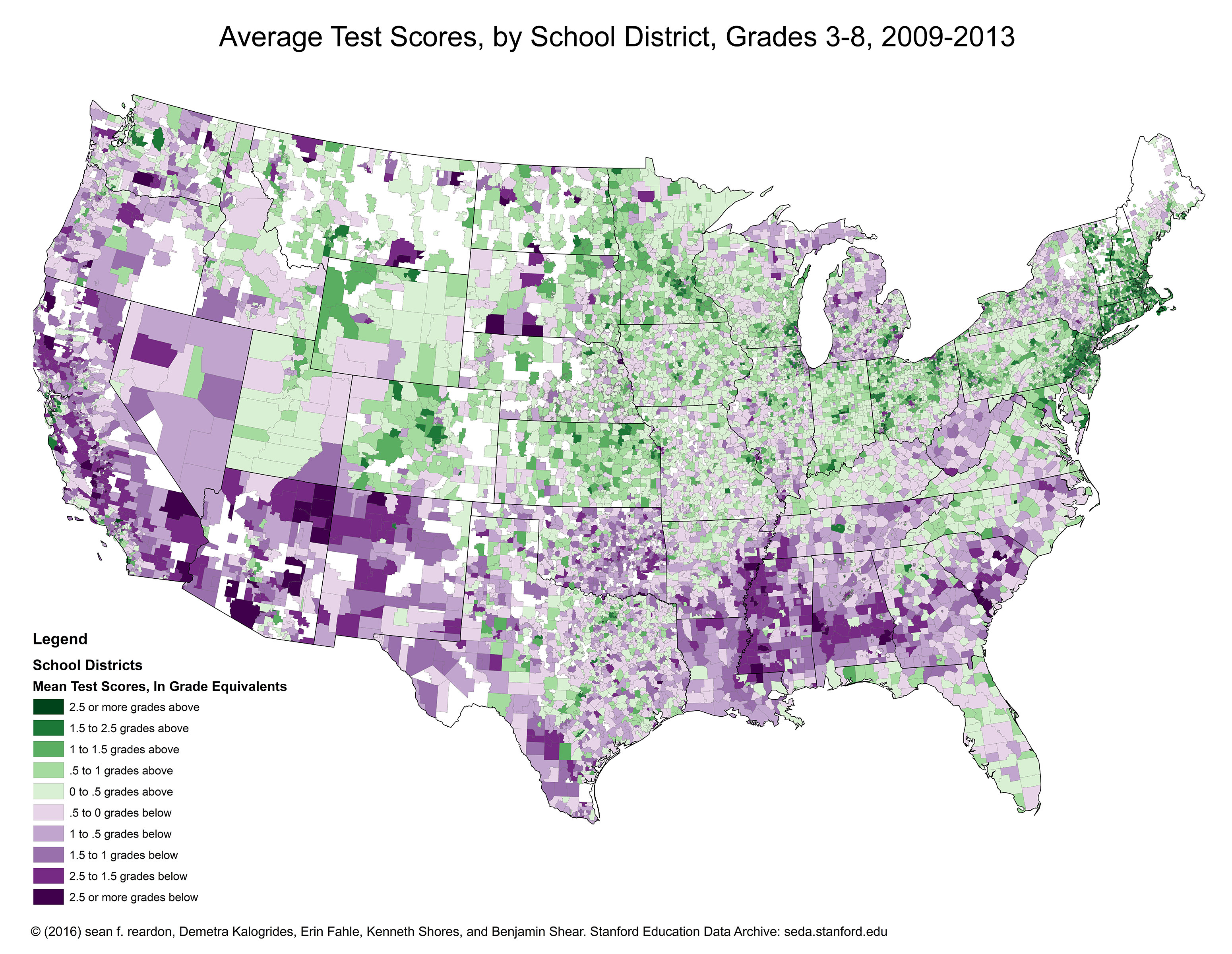# Map - Mean Test Scores, in Grade Equivalents

This map presents Empirical Bayes estimates of district level average achievement. These are centered around zero, so a score of zero means the district is at the average expected level of achievement within the United States. One-unit below zero means that students in the district are one grade level behind the average; one-unit above zero means that students in the district are one grade level above the average. A one-unit change is interpreted as a grade-equivalent change, such as the difference in achievement between grades 3 and 4.